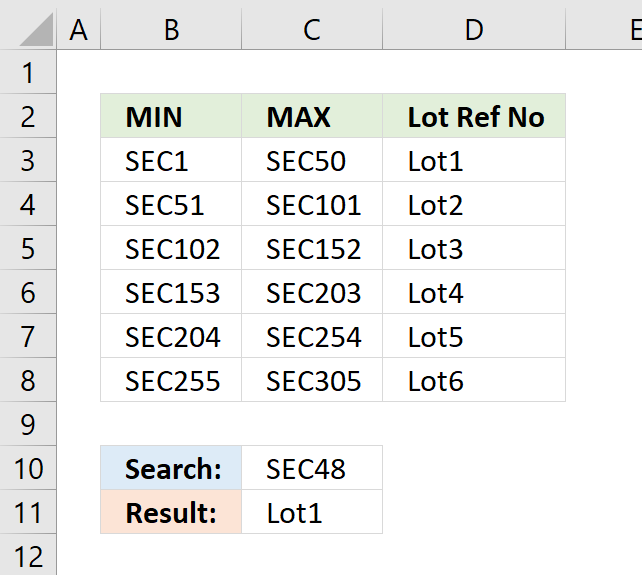Author: Oscar Cronquist Article last updated on October 31, 2018Formula in cell C11:

=LOOKUP(MID(C10, 4, 999)*1, MID(B3:B8, 4, 999)*1, D3:D8)

This formula is an array formula. To enter an array formula, type the formula in a cell then press and hold CTRL + SHIFT simultaneously, now press Enter once. Release all keys.

The formula bar now shows the formula with a beginning and ending curly bracket telling you that you entered the formula successfully. Don't enter the curly brackets yourself.

Explaining formula in cell C11

The MID function returns a given number of characters from a value. This allows us to extract only the numbers from the value.

MID(C10,4,999) becomes MID("SEC48",4,999)

and returns "48".

This is still a text value so in order to use that, we must first convert it to a numerical value.

MID(C10,4,999)*1 becomes "48"*1 and returns 48.

The second argument in the LOOKUP function is the lookup list. This list must also be converted into numbers. I will use the same technique described in the previous step to extract and convert the numbers from cell range B3:B8.

MID(B3:B8,4,999)*1 becomes MID({"SEC1"; "SEC51"; "SEC102"; "SEC153"; "SEC204"; "SEC255"}, 4, 999)*1

becomes {"1";"51";"102";"153";"204";"255"}*1 and returns {1;51;102;153;204;255}

The LOOKUP function matches the lookup value to a list of range values and returns the corresponding value. Remember that the list of values must be sorted in an ascending order for it to work.

LOOKUP(MID(C10,4,999)*1,MID(B3:B8,4,999)*1,D3:D8)

becomes

LOOKUP(48,{1;51;102;153;204;255},{"Lot1"; "Lot2"; "Lot3"; "Lot4"; "Lot5"; "Lot6"})

and returns "Lot1" in cell C11.